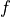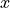# Unit Testing¶

Warning

This document is very outdated.

Aesara relies heavily on unit testing. Its importance cannot be stressed enough!

Unit Testing revolves around the following principles:

• ensuring correctness: making sure that your Op, Type or optimization works in the way you intended it to work. It is important for this testing to be as thorough as possible: test not only the obvious cases, but more importantly the corner cases which are more likely to trigger bugs down the line.
• test all possible failure paths. This means testing that your code fails in the appropriate manner, by raising the correct errors when in certain situations.
• sanity check: making sure that everything still runs after you’ve done your modification. If your changes cause unit tests to start failing, it could be that you’ve changed an API on which other users rely on. It is therefore your responsibility to either a) provide the fix or b) inform the author of your changes and coordinate with that person to produce a fix. If this sounds like too much of a burden… then good! APIs aren’t meant to be changed on a whim!

We use pytest. New tests should generally take the form of a test function, and each check within a test should involve an assertion of some kind.

Note

Tests that check for a lack of failures (e.g. that Exceptions aren’t raised) are generally not good tests. Instead, assert something more relevant and explicit about the expected outputs or side-effects of the code being tested.

## How to Run Unit Tests¶

Mostly pytest aesara/

## Folder Layout¶

Files containing unit tests should be prefixed with the word “test”.

Ideally, every python module should have a unittest file associated with it, as shown below. Unit tests that test functionality of module <module>.py should therefore be stored in tests/<sub-package>/test_<module>.py:

Aesara/aesara/tensor/basic.py
Aesara/tests/tensor/test_basic.py

Aesara/aesara/tensor/elemwise.py
Aesara/tests/tensor/test_elemwise.py


## Test Cases and Methods¶

Unit tests should be grouped “logically” into test cases, which are meant to group all unit tests operating on the same element and/or concept.

Test cases should be functions or classes prefixed with the word “test”.

Test methods should be as specific as possible and cover a particular aspect of the problem. For example, when testing the Dot Op, one test method could check for validity, while another could verify that the proper errors are raised when inputs have invalid dimensions.

Test method names should be as explicit as possible, so that users can see at first glance, what functionality is being tested and what tests need to be added.

## Checking for correctness¶

When checking for correctness of mathematical expressions, the user should preferably compare aesara’s output to the equivalent NumPy implementation.

Example:

import numpy as np
import aesara.tensor as at

def test_dot_validity():
a = at.dmatrix('a')
b = at.dmatrix('b')
c = at.dot(a, b)

c_fn = aesara.function([a, b], [c])

avals = ...
bvals = ...

res = c_fn(avals, bvals)
exp_res = np.dot(self.avals, self.bvals)
assert np.array_equal(res, exp_res)


### Creating an Op Unit Test¶

A few tools have been developed to help automate the development of unit tests for Aesara Ops.

The aesara.gradient.verify_grad() function can be used to validate that the Op.grad() method of your Op is properly implemented. verify_grad() is based on the Finite Difference Method where the derivative of functionat pointis approximated as:verify_grad() performs the following steps:

• approximates the gradient numerically using the Finite Difference Method
• calculate the gradient using the symbolic expression provided in the grad function
• compares the two values. The tests passes if they are equal to within a certain tolerance.

Here is the prototype for the verify_grad() function.

def verify_grad(fun, pt, n_tests=2, rng=None, eps=1.0e-7, abs_tol=0.0001, rel_tol=0.0001):


verify_grad() raises an Exception if the difference between the analytic gradient and numerical gradient (computed through the Finite Difference Method) of a random projection of the fun’s output to a scalar exceeds both the given absolute and relative tolerances.

The parameters are as follows:

• fun: a Python function that takes Aesara variables as inputs, and returns an Aesara variable. For instance, an Op instance with a single output is such a function. It can also be a Python function that calls an Op with some of its inputs being fixed to specific values, or that combine multiple Ops.
• pt: the list of np.ndarrays to use as input values
• n_tests: number of times to run the test
• rng: random number generator used to generate a random vector u, we check the gradient of sum(u*fn) at pt
• eps: stepsize used in the Finite Difference Method
• abs_tol: absolute tolerance used as threshold for gradient comparison
• rel_tol: relative tolerance used as threshold for gradient comparison

In the general case, you can define fun as you want, as long as it takes as inputs Aesara symbolic variables and returns a sinble Aesara symbolic variable:

def test_verify_exprgrad():
def fun(x,y,z):
return (x + at.cos(y)) / (4 * z)**2

x_val = np.asarray([, [1.1], [1.2]])
y_val = np.asarray([0.1, 0.2])
z_val = np.asarray(2)
rng = np.random.default_rng(42)



Here is an example showing how to use verify_grad() on an Op instance:

def test_flatten_outdimNone():
"""
Testing gradient w.r.t. all inputs of an Op (in this example the Op
being used is Flatten, which takes a single input).
"""
a_val = np.asarray([[0,1,2],[3,4,5]], dtype='float64')
rng = np.random.default_rng(42)


Here is another example, showing how to verify the gradient w.r.t. a subset of an Op’s inputs. This is useful in particular when the gradient w.r.t. some of the inputs cannot be computed by finite difference (e.g. for discrete inputs), which would cause verify_grad() to crash.

def test_crossentropy_softmax_grad():
op = at.nnet.crossentropy_softmax_argmax_1hot_with_bias

def op_with_fixed_y_idx(x, b):
# Input y_idx of this Op takes integer values, so we fix them
# to some constant array.
# Although this Op has multiple outputs, we can return only one.
# Here, we return the first output only.
return op(x, b, y_idx=np.asarray([0, 2]))

x_val = np.asarray([[-1, 0, 1], [3, 2, 1]], dtype='float64')
b_val = np.asarray([1, 2, 3], dtype='float64')
rng = np.random.default_rng(42)



Note

Although verify_grad() is defined in aesara.gradient, unittests should use the version of verify_grad() defined in tests.unittest_tools. This is simply a wrapper function which takes care of seeding the random number generator appropriately before calling aesara.gradient.verify_grad()

### makeTester() and makeBroadcastTester()¶

Most Op unittests perform the same function. All such tests must verify that the Op generates the proper output, that the gradient is valid, that the Op fails in known/expected ways. Because so much of this is common, two helper functions exists to make your lives easier: makeTester() and makeBroadcastTester() (defined in module tests.tensor.utils).

Here is an example of makeTester generating testcases for the dot product Op:

import numpy as np

from tests.tensor.utils import makeTester

rng = np.random.default_rng(23098)

TestDot = makeTester(
name="DotTester",
op=np.dot,
expected=lambda x, y: np.dot(x, y),
checks={},
good=dict(
correct1=(rng.random((5, 7)), rng.random((7, 5))),
correct2=(rng.random((5, 7)), rng.random((7, 9))),
correct3=(rng.random((5, 7)), rng.random((7,))),
),
),

In the above example, we provide a name and a reference to the Op we want to test. We then provide in the expected field, a function which makeTester() can use to compute the correct values. The following five parameters are dictionaries which contain:
• checks: dictionary of validation functions (dictionary key is a description of what each function does). Each function accepts two parameters and performs some sort of validation check on each Op-input/Op-output value pairs. If the function returns False, an Exception is raised containing the check’s description.
• bad_build: invalid parameters which should generate an Exception when attempting to build the graph (call to Op.make_node() should fail). Fails unless an Exception is raised.
• bad_runtime: invalid parameters which should generate an Exception at runtime, when trying to compute the actual output values (call to Op.perform() should fail). Fails unless an Exception is raised.
• grad: dictionary containing input values which will be used in the call to verify_grad()
makeBroadcastTester() is a wrapper function for makeTester(). If an inplace=True parameter is passed to it, it will take care of adding an entry to the checks dictionary. This check will ensure that inputs and outputs are equal, after the Op’s perform function has been applied.